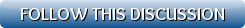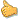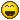[Show all top banners]
 Back to: Kurakani General Discussion Refresh page to view new repliesNormal Distribution (Bell Curve) in C#
 [VIEWED 18076 TIMES] SAVE! for ease of future access.Posted on 03-06-09 12:16 PM     Reply [Subscribe]
Login in to Rate this Post:     0?Hi Gurus,

Have you ever coded Normal Distribution ( Bell Curve) in .NET? I need your help please.

SaagRaSisnuPosted on 03-06-09 12:22 PM     Reply [Subscribe]
Login in to Rate this Post:     0?Lol...Posted on 03-06-09 12:50 PM     Reply [Subscribe]
Login in to Rate this Post:     0?साग सिस्नो जी,

SaagRaSisnuPosted on 03-06-09 1:00 PM     Reply [Subscribe]
Login in to Rate this Post:     0?What do you know about my Level?

AlterEgoPosted on 03-06-09 1:00 PM     Reply [Subscribe]
Login in to Rate this Post:     0?La Dhoti,

Talai gaussian distribution ko class nai banai de ko cha.Download gar ani moj gar.

Or u can create ur own class by using a uniform distribution and then  apply Central Limit theorem to get the Gaussian curve.

AE
Last edited: 06-Mar-09 01:00 PM

hakutheblackPosted on 03-06-09 1:06 PM     Reply [Subscribe]
Login in to Rate this Post:     0?NORMDIST is a statistical function. It has a "nice" formula that is given in the Excel help (go to index, write NORMDIST). C# supports all these functions, just be careful to use the largest possible data types in C# (long, double) otherwise you will lose accuracy.

You can see the help topic with the formula also here:
http://msdn.microsoft.com/library/default.asp?url=/library/en-us/office97/html/sec02.asp

http://support.microsoft.com/default.aspx?kbid=827371&product=xl2003

What normal distribution is:
http://pirate.shu.edu/~wachsmut/Teaching/MATH1101/Testing/distribution.html

SIMPLE C# Implementation

/// <summary>
/// Normal Distribution
/// </summary>
/// <param name="x">The value for which you want the distribution.</param>
/// <param name="mean">The arithmetic mean of the distribution.</param>
/// <param name="deviation">The standard deviation of the distribution.</param>
/// <param name="cumulative">If cumulative is true, functions returns the cumulative distribution, otherwise the function returns the probability mass.</param>
/// <returns>Returns the normal distribution for the specified mean and standard deviation.</returns>
public static double NormalDistribution(double x, double mean, double deviation, bool cumulative)
{
if(cumulative)
return CumulativeDistribution(x, mean, deviation);
else
return NormalDensity(x, mean, deviation);
}

private static double NormalDensity(double x, double mean, double deviation)
{
return Math.Exp(-(Math.Pow((x - mean)/deviation, 2)/2))/Math.Sqrt(2*Math.PI)/deviation;
}

private static double CumulativeDistribution(double x, double mean, double deviation)
{
// TODO: Change the number of iterations (16) for more or less precision.
// You could also change the logic of the recursive function (stop calling
// for more terms, when the values are below a specific threshold for example.
return (ErrorFunction((x - mean)/deviation/Math.Sqrt(2), 0, 16) + 1)/2;
}

private static double ErrorFunction(double x, int iteration, int iterations)
{
double partValue;
partValue = 2/Math.Sqrt(Math.PI)*Math.Pow(-1, iteration)*Math.Pow(x, 2*iteration + 1)/Factorial(iteration)/(2*iteration + 1);

if(iteration==iterations)
return partValue;
else
return ErrorFunction(x, iteration + 1, iterations) + partValue;
}

private static int Factorial(int x)
{
if(x==0)
return 1;
else
return x*Factorial(x-1);
}

hakutheblackPosted on 03-06-09 1:06 PM     Reply [Subscribe]
Login in to Rate this Post:     0?NORMDIST is a statistical function. It has a "nice" formula that is given in the Excel help (go to index, write NORMDIST). C# supports all these functions, just be careful to use the largest possible data types in C# (long, double) otherwise you will lose accuracy.

You can see the help topic with the formula also here:
http://msdn.microsoft.com/library/default.asp?url=/library/en-us/office97/html/sec02.asp

http://support.microsoft.com/default.aspx?kbid=827371&product=xl2003

What normal distribution is:
http://pirate.shu.edu/~wachsmut/Teaching/MATH1101/Testing/distribution.html

SIMPLE C# Implementation

/// <summary>
/// Normal Distribution
/// </summary>
/// <param name="x">The value for which you want the distribution.</param>
/// <param name="mean">The arithmetic mean of the distribution.</param>
/// <param name="deviation">The standard deviation of the distribution.</param>
/// <param name="cumulative">If cumulative is true, functions returns the cumulative distribution, otherwise the function returns the probability mass.</param>
/// <returns>Returns the normal distribution for the specified mean and standard deviation.</returns>
public static double NormalDistribution(double x, double mean, double deviation, bool cumulative)
{
if(cumulative)
return CumulativeDistribution(x, mean, deviation);
else
return NormalDensity(x, mean, deviation);
}

private static double NormalDensity(double x, double mean, double deviation)
{
return Math.Exp(-(Math.Pow((x - mean)/deviation, 2)/2))/Math.Sqrt(2*Math.PI)/deviation;
}

private static double CumulativeDistribution(double x, double mean, double deviation)
{
// TODO: Change the number of iterations (16) for more or less precision.
// You could also change the logic of the recursive function (stop calling
// for more terms, when the values are below a specific threshold for example.
return (ErrorFunction((x - mean)/deviation/Math.Sqrt(2), 0, 16) + 1)/2;
}

private static double ErrorFunction(double x, int iteration, int iterations)
{
double partValue;
partValue = 2/Math.Sqrt(Math.PI)*Math.Pow(-1, iteration)*Math.Pow(x, 2*iteration + 1)/Factorial(iteration)/(2*iteration + 1);

if(iteration==iterations)
return partValue;
else
return ErrorFunction(x, iteration + 1, iterations) + partValue;
}

private static int Factorial(int x)
{
if(x==0)
return 1;
else
return x*Factorial(x-1);
}

hakutheblackPosted on 03-06-09 1:08 PM     Reply [Subscribe]
Login in to Rate this Post:     0?Even Simple

using System;

class NormalDistribution {

static Random rand = new Random();

// returns a normally distributed random number in the range [0..1[
static double NextNormal() {
double x = 0;
for (int i = 0; i < 5; i++) x += rand.NextDouble();
return x / 5;
}

static void Main(string[] arg) {
int[] tab = new int;
// fill tab
for (int i = 0; i < 200; i++) tab[(int)(20 * NextNormal())]++;
// print tab as a histogram
for (int i = 0; i < 20; i++) {
Console.Write("{0,2}: ", i);
for (int j = 0; j < tab[i]; j++) Console.Write("*");
Console.WriteLine();
}
}
}Posted on 03-06-09 1:30 PM     Reply [Subscribe]
Login in to Rate this Post:     0?hakutheblack Ji,

Thank you for your intellectual help. I am sure it will help me.

Also Thanks to AlterEgo for your resource.Posted on 03-09-09 1:44 PM     Reply [Subscribe]
Login in to Rate this Post:     0?How can I get quantity values over the period of time in Bell Curve?

Assumption.

Curve used is the standard normal curve (mean = 0, standard deviation = 1).

End points used for the calculation are three standard deviations from the mean (i.e. since this the standard normal curve this means end points are -3 and +3: this interval captures 99.7% of the area under the curve.

Quantities distributed over N months where N is even will not show any one month with a peak value: months N/2 and N/2+1 will have the same value.Posted on 03-10-09 9:49 AM     Reply [Subscribe]
Login in to Rate this Post:     0?I wish I had cuntinued my C++ class.

bhikhaariPosted on 03-10-09 9:58 AM     Reply [Subscribe]
Login in to Rate this Post:     0?lol

and sorry guys, my level doesn't match with this thread either, may be just parallel (i do perl, unix)